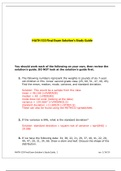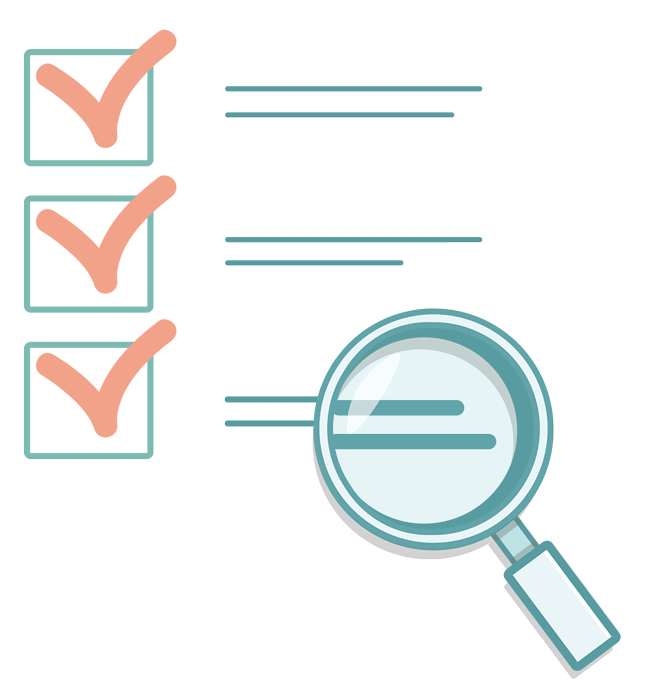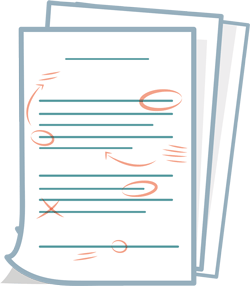MATH 533 Week 8 Final Exam (Solutions Guide) - £22.49   Add to cart

Looking for more study guides & notes about MATH533? Find more study material on our MATH533 overview page

# MATH 533 Week 8 Final Exam (Solutions Guide)

1. Question: The following numbers represent the weights in pounds of six 7-year old children in Mrs. Jones' second grade class (25, 60, 51, 47, 49, 45). Find the mean, median, mode, variance, and standard deviation. 2. Question: If the variance is 846, what is the standard deviation? 3. Question: If we have the following data: 34, 38, 22, 21, 29, 37, 40, 41, 22, 20, 49, 47, 20, 31, 34, 66. Draw a stem and leaf. Discuss the shape of the distribution. 4. Question: For two events, C and D, P(C) = 0.6, P(D)=0.3, and P(C|D) = 0.2. Find P(CD). 5. Question: If a binomial variable has n=34 and p=0.30, find the mean, variance, and standard deviation. 6. Question: A company claims that 61% of consumers know about their product. If the company asks 18 consumers, what is the probability that (a) exactly 10 will say yes, that (b) between 10 and 12 will say yes. 7. Question: If the population has a mean of 58 and a standard deviation of 23.4, what would be the mean and standard deviation of a sampling distribution with a sample size of 22? 8. Question: A restaurant asks 40 customers about their service times. If the population mean service time is 28 minutes with a standard deviation of 5.1 minutes, what is the probability that the mean of the sample service times would be less than 26 minutes? 9. Question: On a dry surface, the braking distance (in meters) of a certain caris a normal distribution with mu = μ= 45.1 m and sigma = σ= 0.5. a. Find the braking distance that represents the 91st percentile. b. Find the probability that the braking distance is less than orequal to 45 m. c. Find the probability that the braking distance is greater than46.8 m. Find the probability that the braking distance is between 45 mand 46.8 m. 10. Question: A sample of 58 days of gas prices has a mean of \$2.75 and a standard deviation of \$0.86. Construct the 95% confidence interval. 11. Question: A drug manufacturer wants to estimate the mean heart rate forpatients with a certain heart condition. Because the condition is rare,the manufacturer can only find 14 people with the condition currentlyuntreated. From this small sample, the mean heart rate is 101 beatsper minute with a standard deviation of 8. a. Find a 99% confidence interval for the true mean heart rate of allpeople with this untreated condition. Show your calculations. b. Interpret this confidence interval, and write a sentence thatexplains it. 12. Question: Determine the minimum required sample size if you want to be80% confident that the sample mean is within 2 units of the populationmean given sigma = 9.4. Assume the population is normallydistributed. 13. Question: A social service worker wants to estimate the true proportion ofpregnant teenagers who miss at least one day of school per week onaverage. The social worker wants to be within 5% of the trueproportion when using a 90% confidence interval. A previous studyestimated the population proportion at 0.21. a. Using this previous study as an estimate for p, what sample sizeshould be used? b. If the previous study was not available, what estimate for pshould be used? 14. Question: A restaurant claims that its speed of service time is less than 15minutes. A random selection of 49 service times was collected, andtheir mean was calculated to be 14.5 minutes. Their standarddeviation is 2.7 minutes. Is there enough evidence to support the claim at alpha = .07? Write the hypotheses, the p-value, conclusion, and implication for the claim. 15. Question: To support their claim that the average speed on their road is more than 25 mph, neighbors collect data on 24 cars on a day. Those cars have a mean speed of 26.7mph and a standard deviation of 3.9mph. Do the neighbors have enough information to support their claim at an alpha of 0.05? Write the hypotheses, the p-value, conclusion, and implication for the claim. 16. Question: A doctor claims that 30% of patients only have a cold. During one month, the doctor finds that 112 of 420 patients only has a cold. At α=0.06, can the doctor support the claim? Write the hypotheses, the p-value, conclusion, and implication for the claim. 17. Question: To test if there was a positive relationship between the dependent and independent variables, a hypothesis test found a t-statistic of 1.43 based on a sample size of 20. Based on this information, what could be concluded about the relationship between these variables using an alpha of 0.05? 18. Question: In a multiple regression with four independent variables and 39 in the sample size, a beta is estimated to be 3.98. Using a standard deviation of this beta of 0.87, find the 95% confidence interval for the beta. 19. Question: Find the regression equation of the following data. X 6 5 7 6 5 6 8 9 4 y 14 33 43 54 21 33 43 24 28 20. Question: To predict the annual rice yield in pounds, we use the equationy-hat= 859 5.76x1 3.82x2,where x1 represents the number of acres planted and where x2 represents the number of acres harvested and where r2 = 0.94. a. Predict the annual yield when 3,200 acres are planted and 3,000 are harvested. b. Interpret the results of r2 value. 21. Question: If a regression model is that y-hat = 5.4x1 - 8.3x2 10, interpret the meaning of the slopes.

Preview 1 out of 8  pages

View exampleHigherEducation

## The benefits of buying summaries with Stuvia:### Guaranteed quality through customer reviews

Stuvia customers have reviewed more than 300,000 summaries. This how you know that you are buying the best documents.### Quick and easy check-out

You can quickly pay through credit card for the summaries. There is no membership needed.### Focus on what matters

Your fellow students write the study notes themselves, which is why the documents are always reliable and up-to-date. This ensures you quickly get to the core!

£ 22.49
• (0)